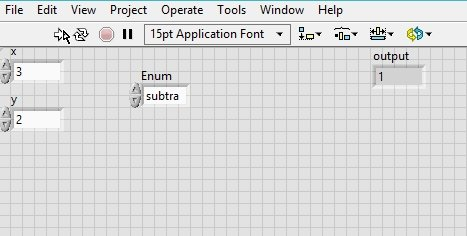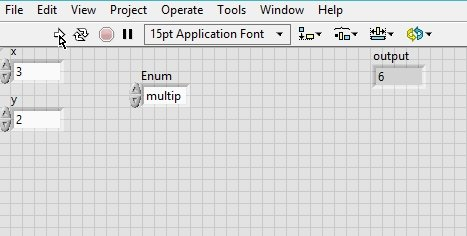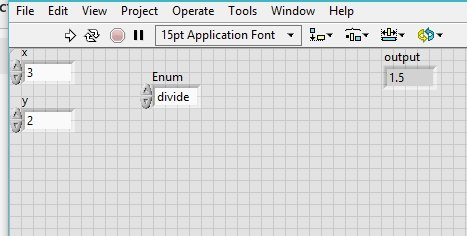# How to design calculator in labview : tutorial 27

In this tutorial I will guide you to design a VI that will help you to do simple mathematical operations. In simple words I will guide you to design a simple calculator that will allow you to add, subtract, multiply and divide two numbers. It will first ask you to enter two digits you want to use as operators, after that you will have to select the operation and the VI will return the output the operation applied to the operands you entered. At the end of the tutorial you are provided with an exercise to do it by yourself, and in the next tutorials I will assume that you have done those exercises and I will not explain the concept regarding them

## Design calculator in labview example

Figure 2: Creating control

Figure 3: Creating indicator

Figure 5: Enum control placement

Figure 6: Edit items window

Figure 7: Adding items in the enum control

Figure 8:Case structure placement

Figure 9: Case structure

Figure 10: Case selector node

Figure 12: Subtract block placement

Figure 13: Subtract case

Figure 15: Multiply block placement

Figure 16: Multiply case

Figure 17: Divide block placement

Figure 18: Divide case

Figure 19: Output with additionFigure 20: Output with subtractionFigure 21: Output with multiplicationFigure 22: Output with division

Exercise:

• Try to evaluate square root and square function as well in this calculator in addition to simple mathematical operations

<< Previous tutorial                                                         Next tutorial>>